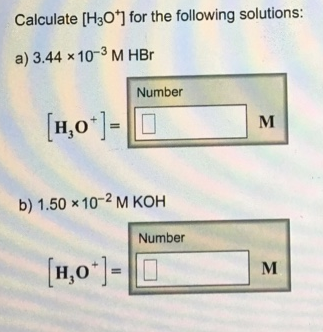# Problem: Calculate [H3O+] for the following solutions:a. 3.44 x 10-3 M HBr[H3O+] = b. 1.50 x 10-2 M KOH[H3O+] =

🤓 Based on our data, we think this question is relevant for Professor Myers' class at NAU.

###### FREE Expert Solution###### Problem Details

Calculate [H3O+] for the following solutions:

a. 3.44 x 10-3 M HBr

[H3O+] =

b. 1.50 x 10-2 M KOH

[H3O+] =